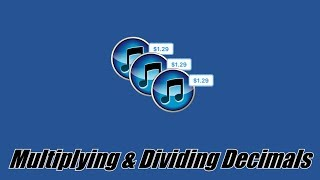# Multiplying and Dividing Decimals | 7.NS.A.2c | 7th Grade Math with Online Resources# Multiplying and Dividing Decimals | 7.NS.A.2c | 7th Grade Math

### DESCRIPTION:

ParksMath explains how to multiply and divide decimals numbers without the use of a calculator. Understanding how to change a decimal number so that it is an integer, makes multiplying and dividing decimals much easier. This quick tutorial will give you thee tools that you need to find the product or quotient of any decimal number.

### OVERVIEW:

Multiplying and Dividing Decimals | 7.NS.A.2c | 7th Grade Math is a educational Book By ParksMath | Todd Parks.It helps students in grades 7.

This page not only allows students and teachers to get information about the book but also find engaging Sample Questions, Videos, Pins, Worksheets, Apps related to the following topics.

Publisher: ParksMath | Todd Parks

### Ratings

Rate this Question?
0

0 Ratings & 0 Reviews

5
0
0
4
0
0
3
0
0
2
0
0
1
0
0EdSearch WebSearch